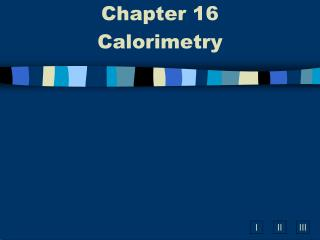DownloadDownload PresentationChapter 16 Calorimetry

# Chapter 16 Calorimetry

Download Presentation## Chapter 16 Calorimetry

- - - - - - - - - - - - - - - - - - - - - - - - - - - E N D - - - - - - - - - - - - - - - - - - - - - - - - - - -
##### Presentation Transcript

1. Chapter 16 Calorimetry

2. Calorimetry • The study of heat flow and heat measurement.

3. Heat Capacity • The amount of heat needed to raise the temperature of an object by 1 Celsius degree.

4. Specific Heat • The heat capacity of 1 gram of a substance • Specific Heat of liquid water is 4.184 J/gºC • 4.184 J = 1 calorie

5. 1 Calorie = 1000 calories = 1 kilocalorie • 1 Food Calorie = 1000 calories

6. Calorimetry Experiments • Determine the heats of reaction (ENTHALPY CHANGES) by making accurate measurements of temperature changes using a calorimeter.

7. Scientists use q to denote measurements made in a calorimeter. • Heat transferred in a reaction is EQUAL, but OPPOSITE in sign to heat absorbed by the surroundings. • qrxn = - qsur

8. HEAT qsur = m x Cp x (Tf –Ti) Temperature change Mass of Water Specific heat of Water

9. Practice Problem: #1 When a 12.8g sample of KCl dissolves in 75.0g of water in a calorimeter, the temperature drops from 31.0ºC to 21.6ºC. Calculate Hfor the process. KCl(s)  K+ (aq) + Cl- (aq) First, calculate qsur and then calculate H.

10. Practice Problem: #1 When a 12.8g sample of KCl dissolves in 75.0g of water in a calorimeter, the temperature drops from 31.0ºC to 21.6ºC. Calculate Hfor the process. KCl (s)  K+ (aq) + Cl-(aq) qsur = m x Cp x (Tf –Ti) qsur = 75.0g x 4.184 J/gºCx (21.6 ºC –31.0 ºC) qsur = -2949.72 J

11. qsur is negative (as expected) based on the temperature drop of the water. qrxn = - qsur = +2949.72 J qrxn represents the heat absorbed due to the reaction of 12.8g KCl. KCl (s)  K+ (aq) + Cl- (aq) Now you must convert the KCl to moles.

12. Convert grams KCl to moles. 1 mol KCl 12.8 g KCl = 0.173 mol KCl 74 g KCl

13. Calculate H for the reaction KCl (s)  K+ (aq) + Cl- (aq) +2949.72 J H = x 1 mol KCl 0.173 mol KCl Coefficient from balanced equation = +17050 J H H Must be positive because it was an endothermic reaction! = H +17.1 kJ

14. Practice Problem: #2 What is the specific heat of aluminum if the temperature of a 28.4 g sample of aluminum is increased by 8.1ºC when 207 J of heat is added? qsur = m x Cp x (Tf –Ti) 207J= 28.4g x Cp x 8.1oC 207J Cp = = 0.90 J/g oC 28.4 g x 8.1 oC